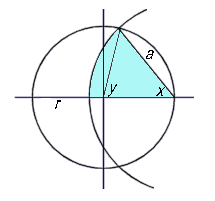### Geometry 101

 This academic exercise is a static problem which goes something like this: A friendly neighbor gave Johnny permission to tie his goat on the perimeter of his circular pasture provided that no more than half of the pasture is grazed. How long should that rope be?pi*a^2*(x/pi) + [pi*r^2*(y/pi) - a*r*sin(x)] = pi*r^2/2 (a/r)^2*x + (pi - 2x) - (a/r)sin(x) = pi/2 2xcos(x)^2 - sin(x)cos(x) - x = -pi/4 The first equation defines the desired surface area of the overlapping circles contoured by the radius r and chord a respectively. A bit of algebra and the angular identity y = pi - 2x produces the second equation. The final equation follows a substitution for the ratio a/r = 2cos(x) which calculates the rope length once the angle x is known. Solving the nonlinear equation for x with, say, Newton-Raphson method, is a quick option for a programmable calculator or a canned PC math package. Here however, we highlight the simple mechanism with which the "solver" interacts with the system to be solved. `````` call nlsq (isol,iev,m,n,x,range_x,tol,fx) if(iev .eq. 1) then fx = (2*x*cos(x) - sin(x))*cos(x) - x + pi/4 iev = 0 endif `````` The solution summarized to a disk file shows x = 54.59 degrees. Inspection of the geometry indicates a solution between pi/4 and pi/3 where the upper bound is determined from the equation x=acos(a/2r) when a = r. The solution is indeed in the right range and Johnny's goat is off to its new pasture.